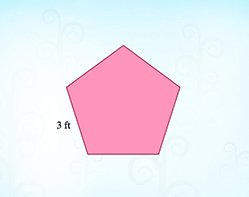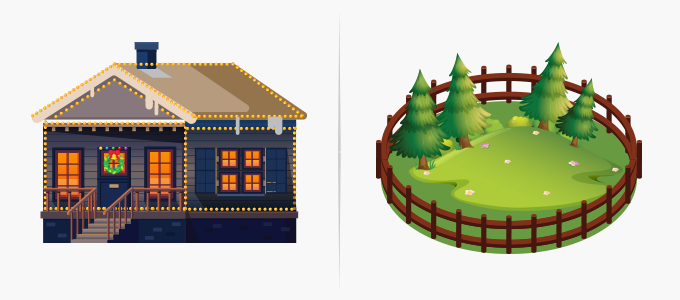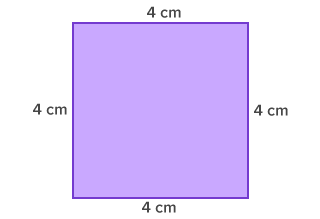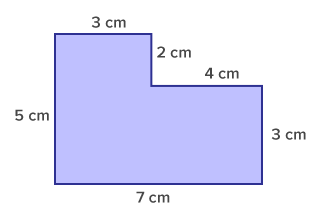# Perimeter - Definition with Examples

The Complete K-5 Math Learning Program Built for Your Child

• 40 Million Kids

Loved by kids and parent worldwide

• 50,000 Schools

Trusted by teachers across schools

• Comprehensive Curriculum

Aligned to Common Core

## Perimeter GamesPerimeter of Regular Figures

Perimeter is the distance around a two-dimensional shape. Add the side lengths to find the perimeter of regular polygons.

Covers Common Core Curriculum 3.MD.8

## What is Perimeter?

In geometry, perimeter can be defined as the path or the boundary that surrounds a shape. It can also be defined as the length of the outline of a shape.The word perimeter has been derived from the Greek word ‘peri’ meaning around, and ‘metron’ which means measure.
Perimeter is the total length of the sides of a two-dimensional shape.

We often find the perimeter when putting up Christmas lights around the house or fencing the backyard garden. Other examples may include finding the total length of the boundary of the soccer field or the length of the crochet or ribbon required to cover the border of a table mat.## How to find perimeter?

We use a ruler to measure length of the sides of a small regular shape. The perimeter is determined by adding the lengths of the sides/edges of the shape.

For small irregular shapes, we can use a string of thread and place it exactly along the boundary of the shape, once. The total length of the string used along the boundary is the perimeter of the shape.The perimeter of all polygons can be determined by adding the lengths of their sides/edges.

 Regular Polygons Irregular PolygonsPerimeter = 4 + 4 + 4 + 4                = 16 cm                OR Perimeter of regular polygons = number of sides x length of one side = 4 x 4  = 16 Perimeter of irregular polygons = 5 + 3 + 2 + 4 + 3 + 7 = 24 cm

Here’s how the perimeter of some common shapes is calculated:

 Polygon name : Polygon Picture/Image : Perimeter Formula : Equilateral TriangleP = 3 x a Scalene TriangleP = a + b + c SquareP = 4 x a RectangleP = 2 ( a + b ) QuadrilateralP = a + b + c + d Regular PentagonP = 5 x a Regular HexagonP = 6 x a Regular OctagonP = 8 x a Regular N-gon A regular n-gon with each side a units long P = n x a , where n is the number of sides and a is the lenght of the sides.

 Fun Facts The Perimeter of a circle is called the circumference. Circumference is calculated using the formula 2π × r .

##Let's sing!

To fence around a movie theatre,
First, Peter found its perimeter.
He added the lengths of all the sides,
and fenced it with flowers big and wide.

##Let's do it!

Instead of giving math practice worksheets on perimeter to your children, give them craft material like craft paper, crayons, glue stick and ask them to create a rectangular Christmas greeting card with a border on all its sides.
You can also show them objects and ask them what’s the perimeter. You can discuss how the perimeter of each of those objects can be calculated.

##Related math vocabulary

Won Numerous Awards & Honors